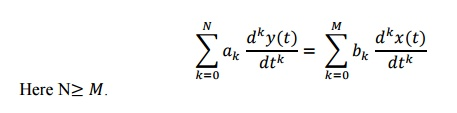Home | | Signals and Systems | Important Short Questions and Answers: Linear Time Invariant, Continuous Time Systems

# Important Short Questions and Answers: Linear Time Invariant, Continuous Time Systems

Signals and Systems - Linear Time Invariant –Continuous Time Systems - Important Short Questions and Answers: Linear Time Invariant, Continuous Time Systems

1. What is the overall impulse response h(t) when two systems with impulse response h1(t) and h2(t) are in parallel and in series?

(or)

State the properties needed for interconnecting LTI systems.

For parallel connection, h(t)=1(t)+2 (t)

For series connection, h(t)= 1(t) ∗ℎ2(t).

2. Write convolution integral of x(t) (or) Define convolution integral of continuous time  systems.3. Check whether the causal system with transfer function H(s) = 1/(s-2) is stable

Here the pole lies at s = 2. Since the pole of causal system does not lie on the left side of jω axis, the system is not stable.

4. The impulse response of the LTI – CT system is giv en as h(t)=e-tu(t). Determine transfer function and check whether the system is causal and stable.

h(t) = e-tu(t)

Taking laplace transform,

H(s) = 1/(s+1)

Here the pole lies at s = -1, i.e. located in left half of s-plane. Hence this system is causal and stable.

5. What are the conditions for a system to be LTI system?

Input and output of an LTI system are related by,6. What is the impulse response of two LTI systems connected in parallel?

If the system are connected in parallel, having responses h1(t)  and h2(t), then their

overall response is given as,

h(t)  = h1(t) + h2(t)

7. Write Nth order differential equation.

The Nth order differential equation can be written as,8. What is the condition for LTI system to be stable?

An LTI system is stable if the impulse response is absolutely integrable.9. What is meant by impulse response of any system?

When the unit impulse function is applied as input to the system, the output is nothing but impulse response h(t). The impulse response is used to study various properties of the system such as causality, stability, dynamicity etc.10. Determine the response of the system with impulse response h(t)= t u(t) for the input x(t)= u(t)

The response is given as,11. State the properties of convolution.

1)          Commutative property:x(t)*h(t) = h(t)*x(t)

2)          Associative property: [x(t)*h1(t)]*h2(t) = x(t)*[h1(t)*h2(t)]

3)          Distributive property:x(t)*h1(t)+x(t)*h2(t) = x(t)*[h1(t)+h2(t)]

12.      What  are  the  three  elementary  operations  in  block  diagram  representation  of

continuous time system?

·     Scalar multiplication

X(t)  --- > y(t) = ax(t)

Study Material, Lecturing Notes, Assignment, Reference, Wiki description explanation, brief detail
Signals and Systems : Linear Time Invariant –Continuous Time Systems : Important Short Questions and Answers: Linear Time Invariant, Continuous Time Systems |